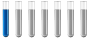Van der Waals Equation - solve for nAuthor Message

NewbieJoined: 26 Feb 2020
Posts: 1# Van der Waals Equation - solve for n

I'm currently having trouble solving for n in the VdW gas equation for a calculator in excel for a variation of gases. I know there is a calculator on the site and I've tried my hand at doing the algebra, but I keep seeming to be just a touch off.

Is there anyone out there that has solved for n in this equation? If so, what is it???

Thanks!Distinguished MemberJoined: 27 Sep 2017
Posts: 353
Location: Berlin, Germanyp = nRT/(V - nb) -n^2 *a/V^2 p ( V - nb) = nRT - n^2*a*(V-nb)/V^2 pV^3 -pnbV^2 = nRTV^2 -n^2aV - n^3ab n^3ab + n^2aV - nRTV^2- pnbV^2 + pV^3 = 0 n^3ab + n^2aV - n( RTV^2+pbV^2) + pV^3 = 0 n^3 + n^2*V/b - n( RTV^2+pV^2)/ab +pV^3/ab =0 This cubic equation is a type of ax^3 + bx^2 + cx+ d = 0 This kind of questions have to be solved with tools of mathematics like curve discussion and differential equation, etc.Display posts from previous: All Posts1 Day7 Days2 Weeks1 Month3 Months6 Months1 Year Oldest FirstNewest FirstPage 1 of 1